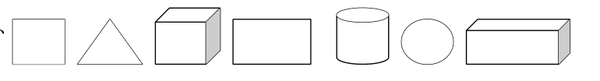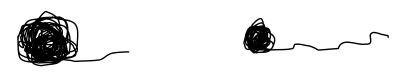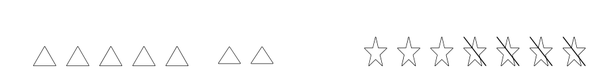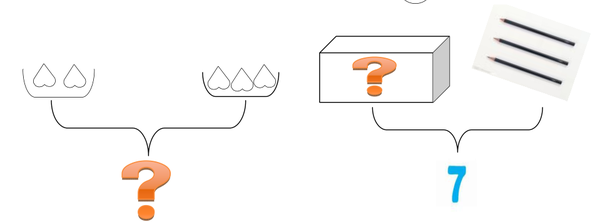# 一年级上册数学期中试卷人教版hanyanyi2021-11-16原创

2-0=    3+0=    0+5=    5+2=    4-4=    4+1=

3+1=    5-3=    7-2=    6-4=    5-4=    7-5=

1.7比2多（ ）4比6少（ ）

2.比5多2的数是（）比7少3的数是（）

3.6比（）多1 5和（）一样多

4、（1）上面一共有（）个图形

（2）从左数圆柱体是第（）个，第2个图形是（）。

（3）把右边3个图形圈起来。

（4）从左边数，把第6个图形涂色。

1.在多的后面画“√”2.在长的后面画“√”

△△△△△△（）

☆☆☆☆☆（）（）（）

3.比一比

☆☆☆☆☆☆○○○○○

▽▽▽▽▽◇◇◇◇◇◇◇

☆比▽比多

▽比☆比少

7-2           3

7-4           4

3+3          5

6-2           6

2+5          1

5-3           2

6-5           0

7-7           7

7-()=4

5-()=3

4+()=7

3+()=6

()-3=2

()+2=7

()-4=2

6+()=6

（）+（）=（）（）-（）=（）

（）+（）=（）（）-（）=（）()[]()=()

()[]()=()

()[]()=()

()[]()=()